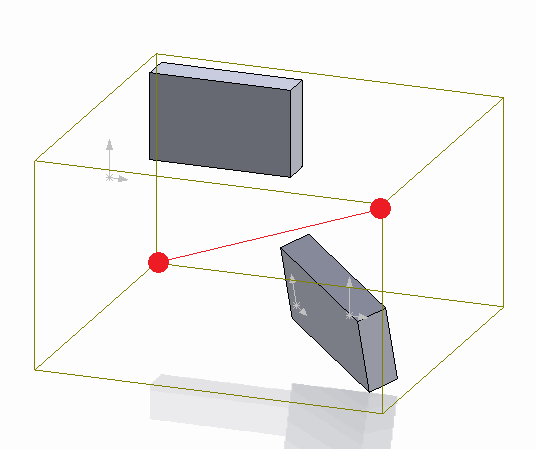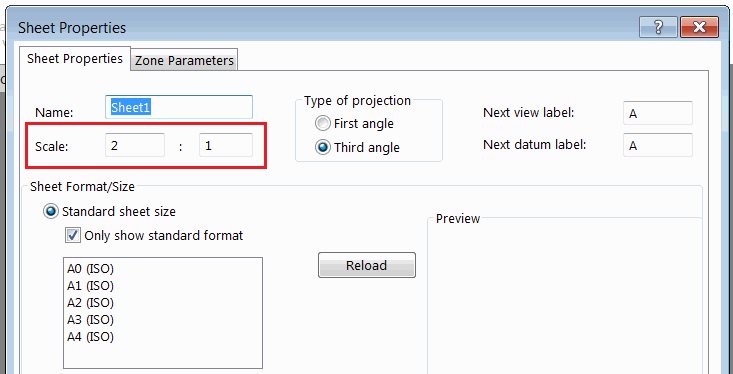# Draw sketch segments in context of the drawing sheet using SOLIDWORKS API

This code example demonstrates how to draw the model bounding box diagonal in the drawing view using SOLIDWORKS API.Bounding box of the assembly

The bounding box coordinate system is extracted from the underlying model of the drawing view. The coordinates are relative to the global coordinate system of the part or the assembly drawing view created from.

In order to properly transform the coordinate into the drawing sheet space it is required to consider the following:

• Drawing view transformation. This can be extracted using the IView::ModelToViewTransform SOLIDWORKS API method.
• Drawing sheet transformation.
• Drawing sheet scaleDrawing sheet scale property

The combination of the above transformation will return the full transformation of the coordinate from the model space into the current sheet space.

When inserting the sketch segments into the drawing sheet it is imported to activate the sheet space by calling the IDrawingDoc::ActivateView SOLIDWORKS API method and passing an empty string as the parameter. Otherwise the entity will be inserted directly into the model space of the view document.

## Running macro

• Open drawing
• Insert view of part or assembly
• Modify view and drawing sheet scale. You can also rotate the view
• Run the macro. As the result the diagonal is drawn in the sheet space representing the bounding box of the underlying model
• Move the view. Note that the created line segment doesn't move with the view which means it was created in the drawing sheet spaceBounding box diagonal in the drawing

```Dim swApp As SldWorks.SldWorks

Sub main()

Set swApp = Application.SldWorks

Dim swDraw As SldWorks.DrawingDoc

Set swDraw = swApp.ActiveDoc

If Not swDraw Is Nothing Then

Dim swView As SldWorks.view
Set swView = swDraw.SelectionManager.GetSelectedObject6(1, -1)

If Not swView Is Nothing Then
DrawBBoxDiagonal swDraw, swView
Else
MsgBox "Please select drawing view"
End If
Else
MsgBox "Please open the drawing document"
End If

End Sub

Sub DrawBBoxDiagonal(draw As SldWorks.DrawingDoc, view As SldWorks.view)

Dim vBox As Variant
Dim swViewTransform As SldWorks.MathTransform

Dim swMathPt As SldWorks.MathPoint
Dim vStartPt As Variant
Dim vEndPt As Variant

vBox = GetViewRefModelBBox(view)
Set swViewTransform = GetViewToSheetTransform(draw, view)

Dim swMathUtils As SldWorks.MathUtility
Set swMathUtils = swApp.GetMathUtility

Dim dPt(2) As Double
dPt(0) = vBox(0): dPt(1) = vBox(1): dPt(2) = vBox(2)

Set swMathPt = swMathUtils.CreatePoint(dPt)
Set swMathPt = swMathPt.MultiplyTransform(swViewTransform)
vStartPt = swMathPt.ArrayData

dPt(0) = vBox(3): dPt(1) = vBox(4): dPt(2) = vBox(5)

Set swMathPt = swMathUtils.CreatePoint(dPt)
Set swMathPt = swMathPt.MultiplyTransform(swViewTransform)
vEndPt = swMathPt.ArrayData

draw.ActivateView ""

draw.ClearSelection2 True

draw.SketchManager.CreateLine vStartPt(0), vStartPt(1), vStartPt(2), vEndPt(0), vEndPt(1), vEndPt(2)

End Sub

Function GetViewRefModelBBox(view As SldWorks.view) As Variant

Dim swRefDoc As SldWorks.ModelDoc2
Set swRefDoc = view.ReferencedDocument

If Not swRefDoc Is Nothing Then
If swRefDoc.GetType() = swDocumentTypes_e.swDocPART Then
Dim swPart As SldWorks.PartDoc
Set swPart = swRefDoc
GetViewRefModelBBox = swPart.GetPartBox(True)
ElseIf swRefDoc.GetType() = swDocumentTypes_e.swDocASSEMBLY Then
Dim swAssy As SldWorks.AssemblyDoc
Set swAssy = swRefDoc
Const BOX_OPTS_DEFAULT As Integer = 0
GetViewRefModelBBox = swAssy.GetBox(BOX_OPTS_DEFAULT)
Else
Err.Raise vbError, "", "Unsupported view document"
End If
Else
Err.Raise vbError, "", "No document attached to view"
End If

End Function

Function GetViewToSheetTransform(draw As SldWorks.DrawingDoc, view As SldWorks.view) As SldWorks.MathTransform

Dim swMathUtils As SldWorks.MathUtility
Dim swSheet As SldWorks.sheet

Set swMathUtils = swApp.GetMathUtility

Set swSheet = view.sheet

Dim vSheetPrps As Variant
vSheetPrps = swSheet.GetProperties

Dim sheetScaleNom As Double
Dim sheetScaleDenom As Double

sheetScaleNom = vSheetPrps(2)
sheetScaleDenom = vSheetPrps(3)

Dim dSheetData(15) As Double
dSheetData(0) = 1: dSheetData(1) = 0: dSheetData(2) = 0: dSheetData(3) = 0
dSheetData(4) = 1: dSheetData(5) = 0: dSheetData(6) = 0: dSheetData(7) = 0
dSheetData(8) = 1: dSheetData(9) = 0: dSheetData(10) = 0: dSheetData(11) = 0
dSheetData(12) = sheetScaleNom / sheetScaleDenom: dSheetData(13) = 0: dSheetData(14) = 0: dSheetData(15) = 0

Dim swSheetTransform As SldWorks.MathTransform
Set swSheetTransform = swMathUtils.CreateTransform(dSheetData)

Set GetViewToSheetTransform = view.ModelToViewTransform.Multiply(swSheetTransform.Inverse())

End Function
```